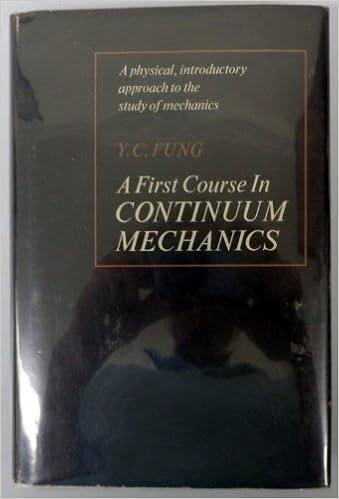## 彭天放得到《硬科技报告》：中国新一代“人造太阳”实现首次放电12月4号，被称为新一代“人造太阳”的核聚变装置，中国环流器二号M装置成功实现了首次放电。这意味着，中国已经自主掌握了大型先进托卡马克装置的设计、建造和运行的相关技术，是我们在可控核聚变领域一项重大的技术进步。换句话说，我们国家距离使用核聚变作为能源发电又近了一步。

- 阅读剩余部分 -

## 彭天放得到《硬科技报告》：中国量子计算原型机“九章”问世- 阅读剩余部分 -

## 《新科学家》专访张锋，纵论基因编辑技术《新科学家》专访张锋，不避争议，坦陈CRISPR的未来前景。张锋对CRISPR技术的未来很乐观，这项技术近期可能有助于我们对抗新冠病毒，长远看来，其应用范围可能会远远超出医学范畴。

- 阅读剩余部分 -

## 标量、矢量和笛卡尔张量的解析定义\begin{equation} x'_i=\beta_{ij}x_j \label{x'x} \end{equation}

\begin{equation} x_i=\beta_{ji}x'_j \label{xx'} \end{equation}

\begin{equation} \Phi(x_1,x_2,x_3)=\Phi'(x'_1,x'_2,x'_3) \label{scalar} \end{equation}

\begin{equation} \begin{cases} \xi'_i(x'_1,x'_2,x'_3)=&\xi_i(x_1,x_2,x_3)\beta_{ik} \\ \xi_i(x'_1,x'_2,x'_3)=&\xi'_i(x_1,x_2,x_3)\beta_{ki} \end{cases} \label{vector} \end{equation}

\begin{equation} \begin{cases} t'_{ij}(x'_1,x'_2,x'_3)=&t_{mn}(x_1,x_2,x_3)\beta_{im}\beta_{jn} \\ t_{ij}(x_1,x_2,x_3)=&t'_{mn}(x'_1,x'_2,x'_3)\beta_{mi}\beta_{nj} \end{cases} \label{tensor} \end{equation}

## 王立铭得到《生命科学50讲》：为什么外星人一定有眼睛？- 阅读剩余部分 -